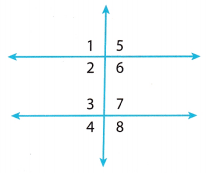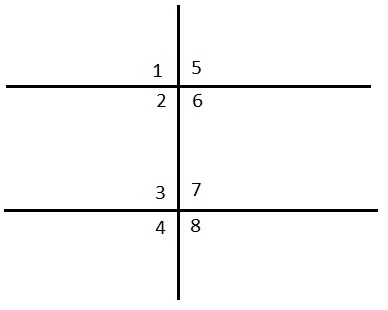# Texas Go Math Grade 8 Lesson 7.1 Answer Key Parallel Lines Cut by a Transversal

Refer to our Texas Go Math Grade 8 Answer Key Pdf to score good marks in the exams. Test yourself by practicing the problems from Texas Go Math Grade 8 Lesson 7.1 Answer Key Parallel Lines Cut by a Transversal.

## Texas Go Math Grade 8 Lesson 7.1 Answer Key Parallel Lines Cut by a Transversal

Explore Activity 1

Parallel Lines and Transversals

A transversal is a line that intersects two lines in the same plane at two different points. Transversal f and lines a and b form eight angles.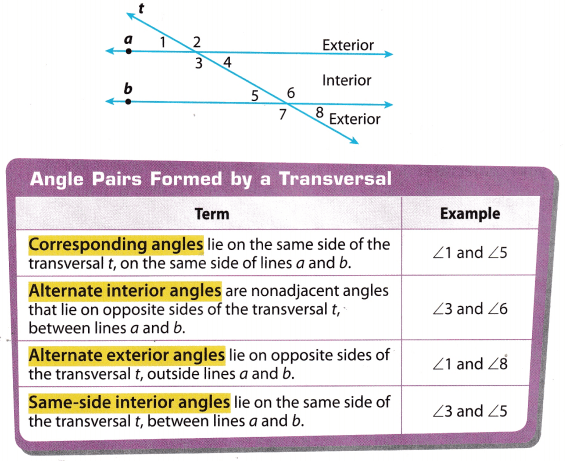Use geometry software to explore the angles formed when a transversal intersects parallel lines.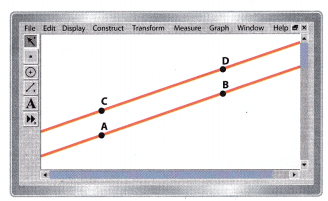A. Construct a line and label two points on the line A and B.
B. Create point C not on $$\overleftrightarrow{A B}$$. Then construct a line parallel to $$\overleftrightarrow{A B}$$ through point C. Create another point on this line and label it D.
C. Create two points outside the two parallel lines and label them E and F. Construct transversal $$\overleftrightarrow{E F}$$. Label the points of intersection G and H.D. Measure the angles formed by the parallel lines and the transversal. Write the angle measures in the table below.
E. Drag point E or point F to a different position. Record the new angle measures in the table.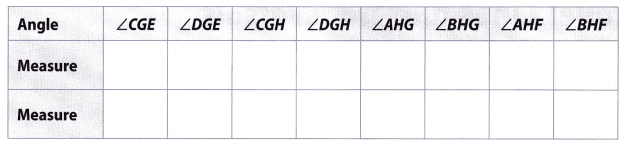Reflect

Make a Conjecture Identify the pairs of angles in the diagram. Then make a conjecture about their angle measures. Drag a point in the diagram to confirm your conjecture.

Question 1.
corresponding angles
Corresponding angles ∠CGE = ∠AHG and ∠DGE = ∠BHG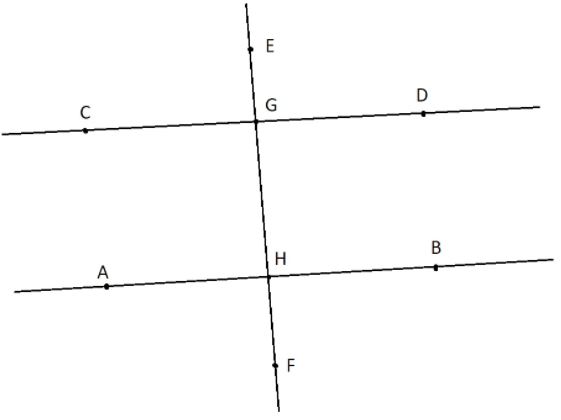Question 2.
alternate interior angles
Alternate interior angles ∠CGH and ∠GHB, and the second pair ∠DGH and ∠AHGQuestion 3.
alternate exterior angles
Alternate exterior angles ∠CGE = ∠BHF and second pair ∠EGD = ∠AHFQuestion 4.
same-side interior angles
Same-side interior angles ∠CGH and angle ∠AHG, and second pair ∠DGH and ∠GHBExplore Activity 2

Justifying Angle Relationships

You can use tracing paper to informally justify your conclusions from the first Explore Activity.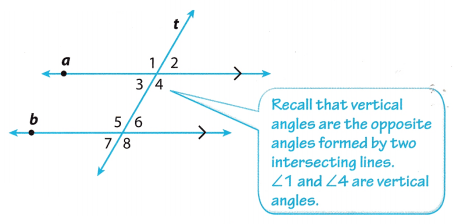Lines a and b are parallel. (The black arrows on the diagram indicate parallel lines.)
A. Trace the diagram onto tracing paper.
B. Position the tracing paper over the original diagram so that ∠1 on the tracing is over ∠5 on the original diagram. Compare the two angles. Do they appear to be congruent?
C. Use the tracing paper to compare all eight angles in the diagram to each other. List all of the congruent angle pairs.

Find each angle measure.Question 5.
m∠GDE = __________
Find m∠GDE
Angles m∠GDE and m∠DEH are vertical angles so
m∠GDE = m∠DEH = 4x°
Angle m∠GDE is supplementary to m∠DEH because they are same-side interior angles
m∠GDE + m∠DEH = 180° …………………… (1)
4x° + 6x° = 180° (Replace m∠GDE with 4x°) and m∠DEH with 6x° ………….. (2) & (3)
10x° = 180° (Combine like terms.) ……………. (4)
x° = $$\frac{1}{2}$$ (Divide both sides with 10) …………… (5)
x = 18° (Simplify) ………….. (6)
m∠GDE = 4x° = (4 ∙ 18)° = 72°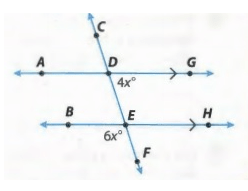Question 6.
m∠BEF = ___________
Find m∠BEF.
Angles m∠GDE and m∠DEH are vertical angles so
m∠GDE = m∠DEH = 4x°
Angle m∠GDE is supplementary to m∠DEH because they are same-side interior angles.
m∠GDE + m∠DEH = 1800 …………. (1)
4x° + 6x° = 180° (RepLace m∠GDE with 4x°) and m∠DEH with 6x° …………… (2) & (3)
10x° = 180° (Combine like terms.) …………….. (4)
x° = $$\frac{180^{\circ}}{10}$$ (Divide both sides with 10) ……………… (5)
x = 18° (Simplify) ……………. (6)
m∠BEF = 6x° = (6 ∙ 18)° = 108°

Question 7.
m∠CDG = ____________
∠BEF is congruent to ∠DEH because they are vertical angles. Therefore,
m∠BEF = m∠DEH = 6x°
∠DEH is supplementary to ∠GDE because they are same-side interior angles. Therefore,
m∠DEH + m∠GDE = 180°
6x° + 4x° = 180°
10x = 180
$$\frac{10 x}{10}=\frac{180}{10}$$
x = 18
∠CDG is congruent to ∠DEH because they are corresponding angles. Therefore,
m∠CDG = m∠DEH = 6x° = (6 ∙ 18)° = 108°

Use the figure for Exercises 1-4. (Explore Activity 1 and Example 1)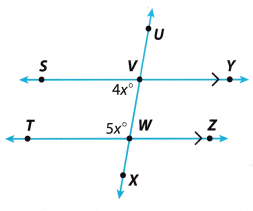Question 1.
∠UVY and ___________ are a pair of corresponding angles.
∠UVY and ∠VWZ are a pair of corresponding angles.

Question 2.
∠WVYand ∠VWT are ____________ angles.
∠WVYand ∠VWT are alternate interior angles.

Question 3.
Find m∠SVW ___________.
∠SVW is supplementary to ∠VWT because they are same-side interior angles. Therefore,
m∠SVW + m∠VWT = 180°
4x° + 5x° = 180°
9x = 180
$$\frac{9 x}{9}=\frac{180}{9}$$
x = 20
m∠SVW = 4x° = (4 ∙ 20)° = 80°

Question 4.
Find m∠VWT. ______________
∠SVW is supplementary to ∠VWT because they are same-side interior angles Therefore.
m∠SVW + m∠VWT = 180°
4x° + 5x° = 180°
9x = 180
$$\frac{9 x}{9}=\frac{180}{9}$$
x = 20
m∠VWT = 5x° = (5 ∙ 20)° = 100°

Question 5.
Vocabulary When two parallel lines are cut by a transversal, ___________ angles are supplementary. (Explore Activity 1)
If two parallel lines are cut by transversal line, interior angles are formed. The pairs of consecutive interior angles are called same-side interior angles which are supplementary Another thing when two parallel lines are cut by a transversal, the pairs of angles on either side of the transversal and inside the two lines are called alternate interior angles which are congruent.

By the description, when two parallel lines are cut by a transversal, same-side interior angles are supplementary.

Essential Question Check-In

Question 6.
What can you conclude about the interior angles formed when two parallel lines are cut by a transversal?
When two parallel lines are cut by a transversal, then four interior angles are formed. These four interior angles could be the alternate interior angles or the same-side interior angles.
Alternate interior angles lie on the alternate side of the transversal and they are congruent.
Same-side interior angles lie on the same side of the transversal which are not adjacent. Same-side interior angles are said to be supplementary.

Vocabulary Use the figure for Exercises 7-10.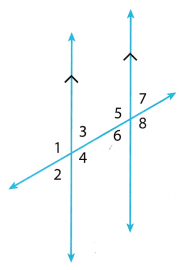Question 7.
Name all pairs of corresponding angles.
Corresponding angles identified in the given diagram are:

• ∠1 and ∠5
• ∠3 and ∠7
• ∠2 and ∠6
• ∠4 and ∠8

Question 8.
Name both pairs of alternate exterior angles.
Alternate exterior angles identified in the given diagram are:

• ∠1 and ∠8
• ∠2 and ∠7

Question 9.
Name the relationship between ∠3 and ∠6.
∠3 and ∠6 are alternate interior angles.

Question 10.
Name the relationship between ∠4 and ∠6.
Angles ∠4 and ∠6 are same-side interior angles.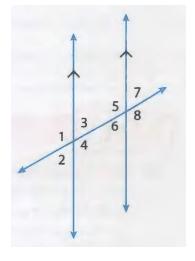Find each angle measure.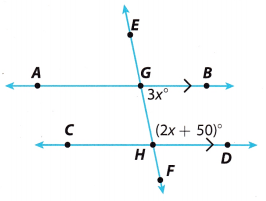Question 11.
m∠AGE when m∠FHD = 30° _________
∠AGE and ∠FHD are alternate exterior angles. Therefore,
m∠AGE = m∠FHD = 30°
m∠AGE = 30°

Question 12.
m∠AGH when m∠CHF = 150° __________
m∠AGH and ∠CHF are corresponding angles. Therefore,
m∠AGH = m∠CHF = 150°
m∠AGH = 150°

Question 13.
m∠CHF when m∠BGE = 110° ___________
∠CHF and ∠BGE are alternate exterior angles. Therefore,
m∠CHF = m∠BGE = 110°
m∠CHF = 110°

Question 14.
m∠CHG when m∠HGA = 120° _____________
∠CHG is supplementary to ∠HGA because they are same-side interior angles. Therefore,
m∠CHG + m∠HGA = 180°
m∠CHG + 120° = 180°
m∠CHG + 120° – 120° = 180° – 120°
m∠CHG = 60°

Question 15.
m∠BGH = _____________∠BGH and ∠GHD are supplementary because they are same-side interior angles. So,
∠BGH + ∠GHD = 180°
3x + (2x + 50)° = 180°
5x = 180° – 50° = 130°
x = $$\frac{130}{5}$$ = 26°
∠BGH = 3x° = 3 × 26° = 78°
∠GHD = (2x + 50)° = (2 × 26° + 50) = 102°

Question 16.
m∠GHD = __________
∠BGH is supplementary to ∠GHD because they are same-side interior angles. Therefore,
m∠BGH + m∠GHD = 180°
3x° + (2x + 50)° = 180°
3x + 2x + 50 – 50 = 180 – 50
5x = 130
$$\frac{5 x}{5}=\frac{130}{5}$$
x = 26
m∠GHD = (2x + 50)° = (2 ∙ 26 + 50)° = (52 + 50)° = 102°
m∠GHD = 102°

Question 17.
The Cross Country Bike Trail follows a straight line where it crosses 350th and 360th Streets. The two streets are parallel to each other. What is the measure of the larger angle formed at the intersection of the bike trail and 360th Street? Explain.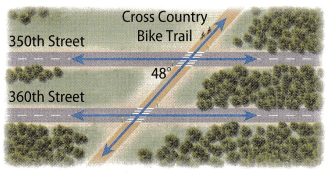For easier calculations, we are going to use the following schema. It is given that m∠3 = 48°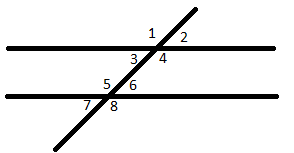The larger angle formed at the intersection of the bike trail and 360th Street is the angle 5 in our schema. ∠5 is supplementary to ∠3 because they are same-side interior angles. Therefore,
m∠5 + m∠3 = 180°
m∠5 + 48°= 180°
m∠5 + 48° – 48° = 180° – 48°
m∠5 = 132°
The larger angle formed at the intersection of the bike trail and 360th Street is 132°

Question 18.
Critical Thinking How many different angles would be formed by a transversal intersecting three parallel lines? How many different angle measures would there be?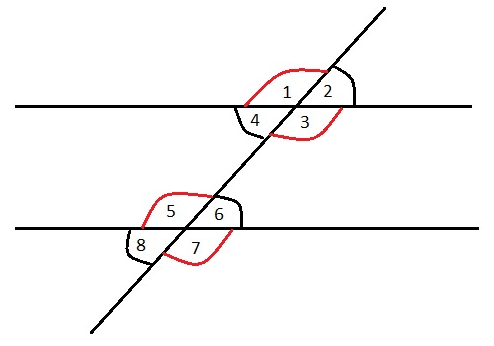As we can see from the above schema, there are 12 different angles formed by a transversal intersecting three parallel lines.
There are 2 different angle measures:

• m∠1 = m∠4 = m∠5 = m∠8 = m∠9 = m∠12
• m∠2 = m∠3 = m∠6 = m∠7 = m∠10 = m∠11

Question 19.
Communicate Mathematical Ideas In the diagram at the right, suppose m∠6 = 125°. Explain how to find the measures of each of the other seven numbered angles.• m∠2 = m∠6 = 125° because ∠2 and ∠6 are corresponding angles.
• m∠3 = m∠2 = 125° because ∠3 and ∠2 are vertical angles.
• m∠7 = m∠3 = 125° because ∠7 and ∠3 are corresponding angles.
• ∠4 is supplementary to ∠6 because they are same-side interior angles. Therefore,

m∠4 + m∠6 = 180°
m∠4 + 125° = 180°
m∠4 + 125° – 125° = 180° – 125°
m∠4 = 55°

• m∠8 = m∠4 = 55° because ∠8 and ∠4 are corresponding angles.
• m∠1 = m∠4 = 55° because ∠1 and ∠4 are vertical angles
• m∠5 = m∠1 = 55° because ∠5 and ∠1 are corresponding angles

H.O.T. Focus on Higher Order Thinking

Question 20.
Draw Conclusions In a diagram showing two parallel lines cut by a transversal, the measures of two same-side interior angles are both given as 3x°. Without writing and solving an equation, can you determine the measures of both angles? Explain. Then write and solve an equation to find the measures.
We are given that the measures of both angles are equal. We also know that the sum of two same-side interior angles is 180°. Therefore, without writing and solving any equation, we divide 180 by 2 and we find that each angle measures 90°.
If we use an equation to find the measures of the angle, we have:
m∠1 + m∠2 = 180°
3x + 3x = 180°
6x = 180°
$$\frac{6 x}{6}=\frac{180}{6}$$
x = 30
m∠1 = m∠2 = 3x = 3 ∙ 30 = 90°

Question 21.
Make a Conjecture Draw two parallel lines and a transversal. Choose one of the eight angles that are formed. How many of the other seven angles are congruent to the angle you selected? How many of the other seven angles are supplementary to your angle? Will your answer change if you select a different angle?
Select angle ∠1, for example. There are 3 congruent angles to ∠1 : ∠3, ∠5, and ∠7
∠3 = ∠1 because they are vertical angles,
∠7 = ∠1 because they are alternate exterior angles.
And, there are 4 supplementary angles: ∠2, ∠4, ∠6 and ∠8
No matter what angle we select, we will always have the same answer – 3 congruent angles, and 4 supplementary.Three of the other seven are congruent to selected angle.

• Four are supplementary.